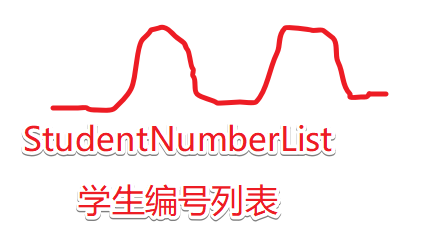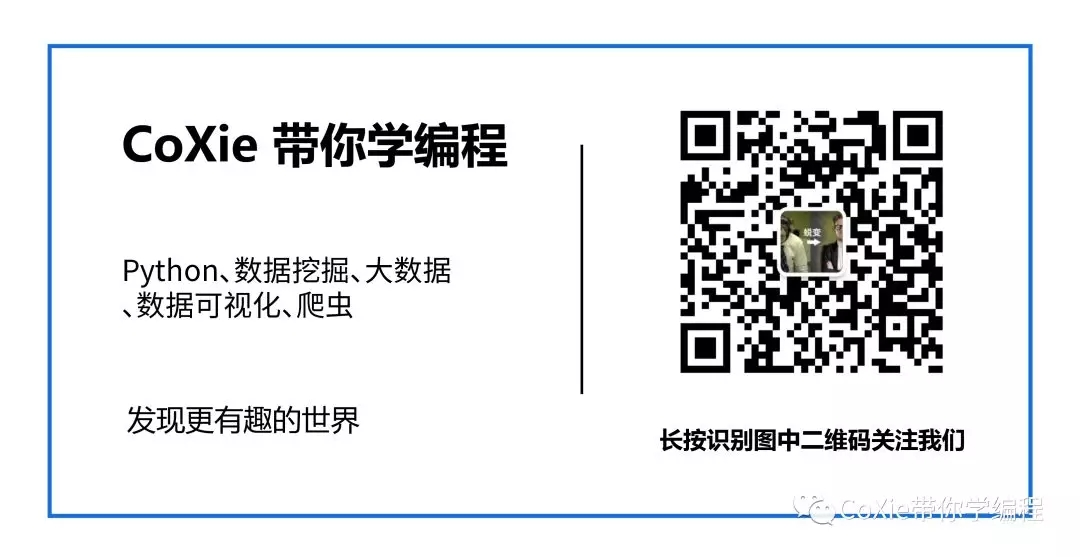# 什么是变量？

3 * 4  = 12

• 一种方法是直接在代码中打出 3 * 4
• 另一种是用一个变量来存储 3 * 4

x = 3
y = 4
z = x * y
print("z = ", z)

z = 12

# 怎么写一个好的变量？

x = "CoXieQqun"
y = "725479218"
z = x + y
print(z)

CoXieQqun725479218

xxx = "CoXieQqun"
xxx = "725479218"

x = "xxxx"
y = "xxxx"
z = x * y 

letters = "CoXieQqun"
Group = "725479218"
my = name + number

# 下划线命名法及驼峰命名法

sutudent  = "CoXie"
number = "01"

studentnumber = "CoXie01"

student_number = "CoXie01"# 结语©️2019 CSDN 皮肤主题: 书香水墨 设计师: CSDN官方博客